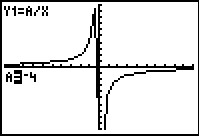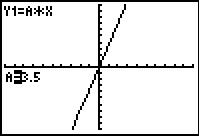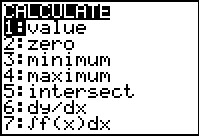# Activities

••• ##### Subject Area

• Math: Algebra II: Rational Expressions
• Math: Algebra II: Equations

• ##### Author9-12

45 Minutes

• ##### Device
• TI-83 Plus Family
• TI-84 Plus
• TI-84 Plus Silver Edition
•TI-84 Plus C Silver Edition
•TI-84 Plus CE

## Constant of Variation#### Activity Overview

Students explore how the constant of variation, k, affects the graph of direct and inverse variations.

#### Key Steps

•Students explore the effects of a, the constant of variation. They will first use the Transformation Graphing app to change a and observe how the direct variation graph changes.

•For the application problem about direct variation, students will use a graph of the function and a point on the graph to determine the amount of money the employee will make for a certain number of hours.

•Students will investigate the effect of a on the graph of an inverse variation using the left and right arrow keys.